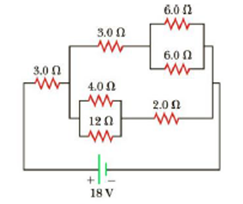Chapter 18, Problem 15P

Chapter
Section
Textbook Problem

Find the current in the 12-Ω resistor in Figure P18.15.Figure P18.15

To determine
The current passing through the 12.0Ω resistor from the figure 1.

Explanation

Given Info: The top of the two resistors across the point c and d connected in parallel combinations are 6.0Ω and 6.0Ω , and the bottom of the two resistors across the point b and e connected in parallel combinations are 4.0Ω and 12.0Ω , The top of the two resistors across the point c connected in series combinations are 3.0Ω and 3.0Ω , and the bottom of the two resistors across the point e connected in series combinations are 3.0Ω and 2.0Ω , The two resistors across the point b and d connected in parallel combinations are 6.0Ω and 5.0Ω , and the resistors across the point a and b connected in series combinations are 3.0Ω and 2.0Ω , The potential difference across the point a and d is ΔVad=18V , resistance across the point b and d is Rbd=2.73Ω and resistance across the point a and d is Rad=5.73Ω , The potential difference across the point b and d is ΔVbd=8.5V , and the resistors is 3.0Ω and 2.0Ω .

Explanation:

The resistors in the circuit can be combined in the stages shown below:

Formula to calculate the equivalent resistance of the two resistors connected in parallel combination is,

1Rp1=1R6+1R6

• Rp1 is the equivalent resistance of the two resistors connected in parallel,
• R6 is the resistor,

Substitute 6.0Ω for R6 to find Rp1 .

1Rp1=16.0Ω+16.0ΩRp1=6.0Ω2=3.0Ω

Thus, the equivalent resistance of the two resistors connected in parallel is Rp1=3.0Ω .

Formula to calculate the equivalent resistance of the two resistors connected in second parallel combination is,

1Rp2=1R4+1R12

• Rp2 is the equivalent resistance of the two resistors connected in second parallel,
• R4 and R12 is the resistors,

Substitute 4.0Ω for R4 and 12.0Ω for R12 to find Rp2 .

1Rp2=14.0Ω+112.0ΩRp2=12.0Ω4=3.0Ω

Thus, the equivalent resistance of the two resistors connected in second parallel is Rp2=3.0Ω .

Formula to calculate the equivalent resistance of the two resistors connected in first series combination is,

Rs1=R3+R3

• Rs1 is the equivalent resistance of the two resistors connected in series,
• R3 is the resistor,

Substitute 3.0Ω for R3 to find Rs1 .

Rs1=3.0Ω+3.0Ω=6.0Ω

Thus, the equivalent resistance of the two resistors connected in series is Rs1=6.0Ω .

Formula to calculate the equivalent resistance of the two resistors connected in second series combination is,

Rs2=R3+R2

• Rs2 is the equivalent resistance of the two resistors connected in second series combination,
• R3 and R2 is the resistors,

Substitute 3.0Ω for R3 and 2.0Ω for R2 to find Rs2 .

Rs2=3.0Ω+2.0Ω=5.0Ω

Thus, the equivalent resistance of the two resistors connected in second series is Rs2=5.0Ω .

Formula to calculate the equivalent resistance of the two resistors connected in parallel combination is,

1Rp=1R6+1R5

• Rp is the equivalent resistance connected in parallel,
• R6 and R5 is the resistors,

Substitute 6.0Ω for R6 and 5.0Ω for R5 to find Rp

Still sussing out bartleby?

Check out a sample textbook solution.

See a sample solution

The Solution to Your Study Problems

Bartleby provides explanations to thousands of textbook problems written by our experts, many with advanced degrees!

Get Started

Complete the following table:

Chemistry & Chemical Reactivity

What are sister chromatids?

Human Heredity: Principles and Issues (MindTap Course List)

Draw structural formulas for cis- and trans-1, 2-dimethylcyclohexane.

Chemistry for Today: General, Organic, and Biochemistry